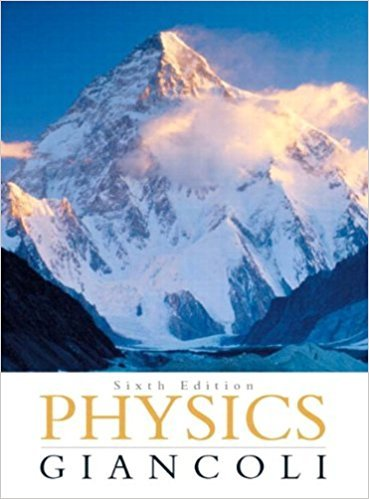×
×

# Solved: On an ice rink, two skaters of equal mass grabISBN: 9780321569837 99

## Solution for problem 68GP Chapter 5

Physics: Principles with Applications | 6th Edition

• Textbook Solutions
• 2901 Step-by-step solutions solved by professors and subject experts
• Get 24/7 help from StudySoup virtual teaching assistantsPhysics: Principles with Applications | 6th Edition

4 5 1 315 Reviews
25
1
Problem 68GP

On an ice rink, two skaters of equal mass grab hands and spin in a mutual circle once every 2.5 s. If we assume their arms are each 0.80 m long and their individual masses are 60.0 kg, how hard are they pulling on one another?

Step-by-Step Solution:

Solution 68GP

Step 1 of 2:

Consider the two ice skaters spin in a mutual circle of radius r and both have same mass m. The time period is T. We are going to find the force on one another.

The mass of one person m = 60 kg

The time period T = 2.5 s

The radius of the circle r = 0.80 m

Step 2 of 2

##### ISBN: 9780321569837

The full step-by-step solution to problem: 68GP from chapter: 5 was answered by , our top Physics solution expert on 09/09/17, 04:43AM. Physics: Principles with Applications was written by and is associated to the ISBN: 9780321569837. The answer to “On an ice rink, two skaters of equal mass grab hands and spin in a mutual circle once every 2.5 s. If we assume their arms are each 0.80 m long and their individual masses are 60.0 kg, how hard are they pulling on one another?” is broken down into a number of easy to follow steps, and 46 words. Since the solution to 68GP from 5 chapter was answered, more than 441 students have viewed the full step-by-step answer. This full solution covers the following key subjects: another, arms, assume, circle, equal. This expansive textbook survival guide covers 33 chapters, and 4141 solutions. This textbook survival guide was created for the textbook: Physics: Principles with Applications, edition: 6.

Unlock Textbook Solution